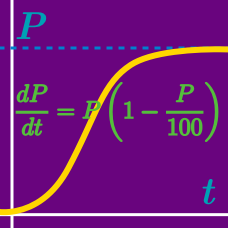Calculus

# Logistic Differential Equations

Given the logistic differential equation $\frac{dP}{dt} = 3 P \left( 1 - \frac{P}{600}\right),$ and $P(0)=P_{0} = 17,$ what time $t$ satisfies $P(t)=100?$

Let $P_0$ denote the initial value $P(0)$ of the function $P(t).$ Which of the following functions satisfy $\frac{dP}{dt} = 5 P \left( 1 - \frac{P}{8}\right)?$

Consider the logistic differential equation $\frac{dP}{dt} = -\ln 2 \times P \left( 1 - \frac{P}{5}\right).$ If $P(4) = 28,$ what is the value of $P(0)$?

Given the logistic differential equation $\frac{dP}{dt} = -\ln 2 \times P \left( 1 - \frac{P}{18}\right),$ if $P(0)=P_{0} = 14,$ what is the value of $P(4)?$

Given the logistic differential equation $\displaystyle{ \frac{dP}{dt} = -2 P \left( 1 - \frac{P}{21}\right)},$ if $P(0)=P_{0} = 18,$ what is the value of $P(\ln3)?$

×